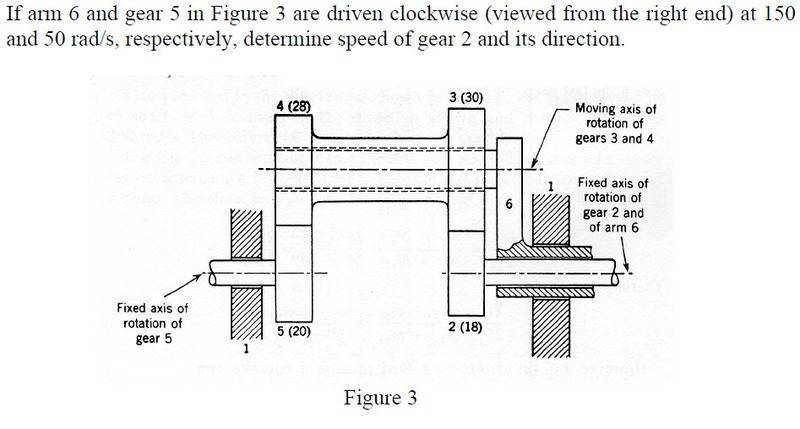# Solving a Complex Math Problem - Need Help!

• Icetray

#### IcetrayThe attempt at a solution

$\frac{n2 - n6}{n5 - n6}$ = $\frac{- 18}{30}$$\frac{-28}{20}$

The answer is -30.9 rad/s (clockwise). The problem looks rather simple and I'm really not sure what I'm doing wrong.

Also if anyone knows where I can get more worked examples on these kinds of problems I'd really appreciate it!

As in the previous problem, consider the frame co-rotating with arm 6. In this frame, $$n_2' = n_2 - n_6 \\ n_5' = n_5 - n_6$$ Then you can easily relate $n_2'$ and $n_5'$ in this frame, and then convert to $n_2$, $n_5$ and $n_6$.